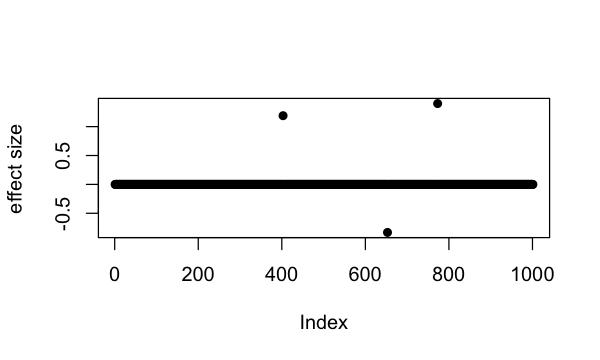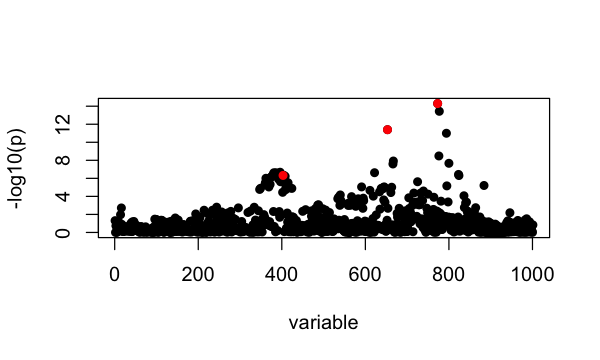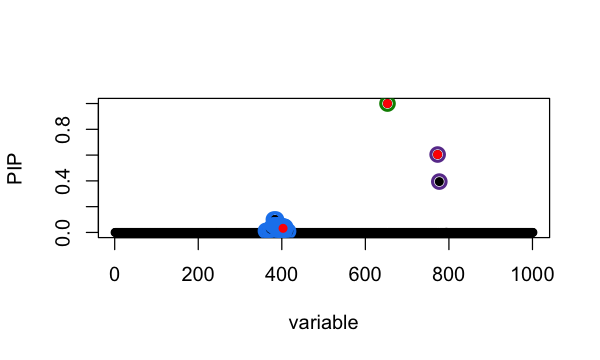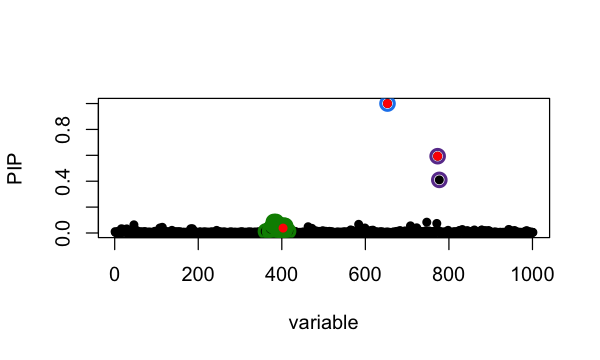Fine-mapping example

2021-11-12

This vignette demonstrates susieR in the context of genetic fine-mapping. We use simulated data of expression level of a gene ($$y$$) in $$N \approx 600$$ individuals. We want to identify with the genotype matrix $$X_{N\times P}$$ ($$P=1001$$) the genetic variables that causes changes in expression level.

The simulated data set is simulated to have exactly 3 non-zero effects.

library(susieR)
set.seed(1)

The data-set

data(N3finemapping)
attach(N3finemapping)
# The following objects are masked from N3finemapping (pos = 3):
#
#     allele_freq, chrom, pos, residual_variance, true_coef, V, X, Y

The loaded dataset contains regression data $$X$$ and $$y$$, along with some other relevant properties in the context of genetic studies. It also contains the “true” regression coefficent the data is simulated from.

Notice that we’ve simulated 2 sets of $$Y$$ as 2 simulation replicates. Here we’ll focus on the first data-set.

dim(Y)
#  574   2

Here are the 3 “true” signals in the first data-set:

b <- true_coef[,1]
plot(b, pch=16, ylab='effect size')which(b != 0)
#  403 653 773

So the underlying causal variables are 403, 653 and 773.

Simple regression summary statistics

univariate_regression function can be used to compute summary statistics by fitting univariate simple regression variable by variable. The results are $$\hat{\beta}$$ and $$SE(\hat{\beta})$$ from which z-scores can be derived. Again we focus only on results from the first data-set:

sumstats <- univariate_regression(X, Y[,1])
z_scores <- sumstats$betahat / sumstats$sebetahat
susie_plot(z_scores, y = "z", b=b)Fine-mapping with susieR

For starters, we assume there are at most 10 causal variables, i.e., set L = 10, although SuSiE is robust to the choice of L.

The susieR function call is:

fitted <- susie(X, Y[,1],
L = 10,
verbose = TRUE)
#  "objective:-1380.57545244487"
#  "objective:-1377.4866091747"
#  "objective:-1375.85777210115"
#  "objective:-1375.80892303931"
#  "objective:-1370.33949333171"
#  "objective:-1370.19677276994"
#  "objective:-1370.10919739202"
#  "objective:-1370.10918017469"
#  "objective:-1370.10901872278"

Credible sets

By default, we output 95% credible set:

The 3 causal signals have been captured by the 3 CS reported here. The 3rd CS contains many variables, including the true causal variable 403. The minimum absolute correlation is 0.86.

If we use the default 90% coverage for credible sets, we still capture the 3 signals, but “purity” of the 3rd CS is now 0.91 and size of the CS is also a bit smaller.

Posterior inclusion probabilities

Previously we’ve determined that summing over 3 single effect regression models is approperate for our application. Here we summarize the variable selection results by posterior inclusion probability (PIP):The true causal variables are colored red. The 95% CS identified are circled in different colors. Of interest is the cluster around position 400. The true signal is 403 but apparently it does not have the highest PIP. To compare ranking of PIP and original z-score in that CS:

Choice of priors

Notice that by default SuSiE estimates prior effect size from data. For fine-mapping applications, however, we sometimes have knowledge of SuSiE prior effect size since it is parameterized as percentage of variance explained (PVE) by a non-zero effect, which, in the context of fine-mapping, is related to per-SNP heritability. It is possible to use scaled_prior_variance to specify this PVE and explicitly set estimate_prior_variance=FALSE to fix the prior effect to given value.

In this data-set, SuSiE is robust to choice of priors. Here we set PVE to 0.2, and compare with previous results:which largely remains unchanged.